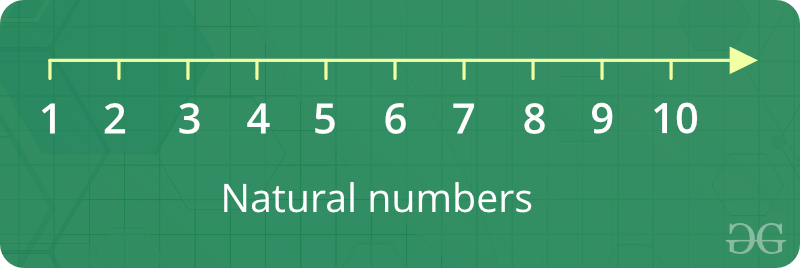Related Articles

# Natural Numbers

• Difficulty Level : Easy
• Last Updated : 18 Aug, 2021

Counting numbers like 1, 2, 3, 4, 5, 6 … Basically, all integers greater than 0 are natural numbers.1. They are whole numbers (called integers), and never less than zero (i.e. positive numbers)
2. The next possible natural number can be found by adding 1 to the current natural number
3. The natural numbers are the ordinary numbers, 1, 2, 3, etc., with which we count.
4. The number zero is sometimes considered to be a natural number. Not always because no one counts starting with zero, 0, 1, 2, 3.
5. GCD of all other natural numbers with a prime is always one.
6. The natural numbers can be defined formally by relating them to sets. Then, zero is the number of elements in the empty set; 1 is the number of elements in the set containing one natural number; and so on.

How to print sum of n natural Numbers?

Using Recursion

Given a number n, find sum of first n natural numbers. To calculate the sum, we will use a recursive function recur_sum().

Examples :

```Input : 3
Output : 6
Explanation : 1 + 2 + 3 = 6

Input : 5
Output : 15
Explanation : 1 + 2 + 3 + 4 + 5 = 15```

## C++

 `// C++ program to find the``// sum of natural numbers up``// to n using recursion``#include ``using` `namespace` `std;` `// Returns sum of first``// n natural numbers``int` `recurSum(``int` `n)``{``    ``if` `(n <= 1)``        ``return` `n;``    ``return` `n + recurSum(n - 1);``}` `// Driver code``int` `main()``{``    ``int` `n = 5;``    ``cout << recurSum(n);``    ``return` `0;``}`

## Java

 `// Java program to find the``// sum of natural numbers up``// to n using recursion``import` `java.util.*;``import` `java.lang.*;` `class` `GFG``{` `    ``// Returns sum of first``    ``// n natural numbers``    ``public` `static` `int` `recurSum(``int` `n)``    ``{``        ``if` `(n <= ``1``)``            ``return` `n;``        ``return` `n + recurSum(n - ``1``);``    ``}``    ` `    ``// Driver code``    ``public` `static` `void` `main(String args[])``    ``{``        ``int` `n = ``5``;``        ``System.out.println(recurSum(n));``    ``}``}`

## Python

 `# Python code to find sum``# of natural numbers upto``# n using recursion` `# Returns sum of first``# n natural numbers``def` `recurSum(n):``    ``if` `n <``=` `1``:``        ``return` `n``    ``return` `n ``+` `recurSum(n ``-` `1``)` `# Driver code``n ``=` `5``print``(recurSum(n))`

## C#

 `// C# program to find the``// sum of natural numbers``// up to n using recursion``using` `System;` `class` `GFG``{` `    ``// Returns sum of first``    ``// n natural numbers``    ``public` `static` `int` `recurSum(``int` `n)``    ``{``        ``if` `(n <= 1)``            ``return` `n;``        ``return` `n + recurSum(n - 1);``    ``}``    ` `    ``// Driver code``    ``public` `static` `void` `Main()``    ``{``        ``int` `n = 5;``        ``Console.WriteLine(recurSum(n));``    ``}``}`

## PHP

 ``

## Javascript

 ``

Output :

```15
```

Using Loop

A simple solution is to do following.

```1) Initialize : sum = 0
2) Run a loop from x = 1 to n and
do following in loop.
sum = sum + x  ```

## C++

 `// CPP program to find sum of first``// n natural numbers.``#include``using` `namespace` `std;` `// Returns sum of first n natural``// numbers``int` `findSum(``int` `n)``{``   ``int` `sum = 0;``   ``for` `(``int` `x=1; x<=n; x++)``     ``sum = sum + x;``   ``return` `sum;``}` `// Driver code``int` `main()``{``  ``int` `n = 5;``  ``cout << findSum(n);``  ``return` `0;``}`

## Java

 `// JAVA program to find sum of first``// n natural numbers.``import` `java.io.*;` `class` `GFG{` `    ``// Returns sum of first n natural``    ``// numbers``    ``static` `int` `findSum(``int` `n)``    ``{``        ``int` `sum = ``0``;``        ``for` `(``int` `x = ``1``; x <= n; x++)``            ``sum = sum + x;``        ``return` `sum;``    ``}` `    ``// Driver code``    ``public` `static` `void` `main(String args[])``    ``{``        ``int` `n = ``5``;``        ``System.out.println(findSum(n));``    ``}``}`

## Python

 `# PYTHON program to find sum of first``# n natural numbers.` `# Returns sum of first n natural``# numbers``def` `findSum(n) :``    ``sum` `=` `0``    ``x ``=` `1``    ``while` `x <``=``n :``        ``sum` `=` `sum` `+` `x``        ``x ``=` `x ``+` `1``    ``return` `sum`  `# Driver code` `n ``=` `5``print` `findSum(n)`

## C#

 `// C# program to find sum of first``// n natural numbers.``using` `System;` `class` `GFG{` `    ``// Returns sum of first n natural``    ``// numbers``    ``static` `int` `findSum(``int` `n)``    ``{``        ``int` `sum = 0;``        ``for` `(``int` `x = 1; x <= n; x++)``            ``sum = sum + x;``        ``return` `sum;``    ``}` `    ``// Driver code``    ``public` `static` `void` `Main()``    ``{``        ``int` `n = 5;``        ``Console.Write(findSum(n));``    ``}``}`

## PHP

 ``

## Javascript

 ``

Output :

`15`

Using Sum of n terms formula

Formula for finding sum of n natural numbers is given by n*(n+1)/2 which implies if the formula is used the program returns output faster than it would take iterating over loop or recursion. Time complexity is O(1).